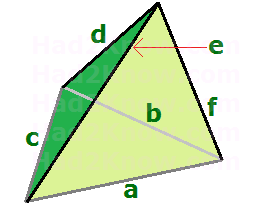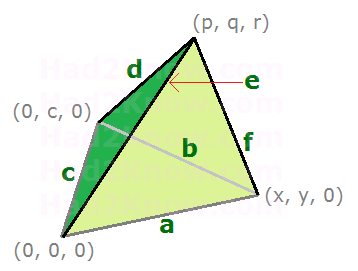# Tetrahedral Volume and Surface Area from Edge Lengths

Tetrahedron Volume Calculator
a =

b =

c =
d =

e =

f =

In geometry, a tetrahedron is 3-dimensional solid figure with 4 vertices, 6 edges, and 4 triangular faces. You can orient a tetrahedron so that it is a triangular pyramid with one triangular face as the base, and the other three triangular faces forming the sides of the pyramid.

You can label the edges of the tetrahedron so that the edges at the base are a, b, and c, while the rising edges are d (opposite a), e (opposite b), and f (opposite c). See figure below:Given the lengths of a, b, c, d, e, and f, you can determine the volume and surface area of the tetrahedron. Apply the equations and geometric principles below, or use the calculator above to find the surface area and volume.

### Surface Area

The surface area of a tetrahedron is the sum of the areas of the triangular faces. Heron's formula gives the area of a triangle when you input the lengths of the sides. Given triangular edge lengths of x, y, and z, the area function H(x, y, z) is defined by the expression

H(x, y, z) = 0.25√2x²y² + 2x²z² + 2y²z² - x4 - y4 - z4

Therefore, the surface area of the entire tetrahedron is

H(a, b, c) + H(a, e, f) + H(b, d, f) + H(c, d, e)

### Volume

The easiest way to compute the volume of a tetrahedron is to find the determinant of a matrix whose entries are given by the coordinates of the vertices. Luckily, you can figure out all four vertex coordinates from the edges. The first step is to fix one vertex at (0, 0, 0) and place another on the y-axis. See figure below:The other two vertices are labeled (x, y, 0) and (p, q, r), where x, y, p, q, and r depend on a, b, c, d, e, and f.

The coordinate y can be computed with the formula

y = (a² + c² - b²)/(2c),

from which follows x = √a² - y², since a is the hypotenuse of a right triangle with legs x and y. Next, q can be calculated with the formula

q = (c² + e² - d²)/(2c),

from which follows

p = (a² + e² - f² - 2qy)/(2x) and
r = √e² - q² - p²

With the coordinates determined, you can use the vertex formula for tetrahedral volume.